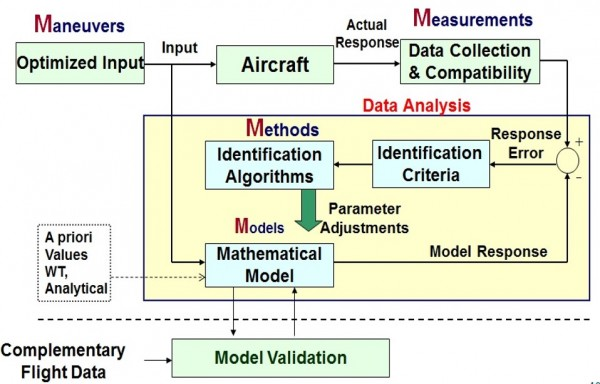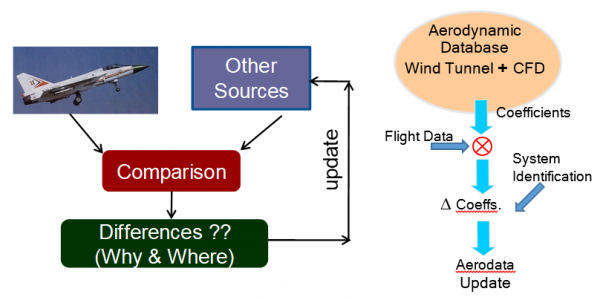# PARAMETER ESTIMATION TECHNIQUES IN TIME DOMAIN AND FREQUENCY DOMAIN#### Parameter Estimation Techniques in time domain and frequency domain

Output Error Method, Filter Error method, Extended Kalman Filter, Feed Forward Neural Networks and Discrete Fourier Transform methods for Identification of Aerodynamic Derivatives, Flight Path Reconstruction, aerodatabase validation and update using incremental models based on coefficient level matching approach.

System Identification Techniques are used for Aerodatabase validation work for Light Combat Aircraft, Mirage-2000 FOC Upgrade, HTT-40 and SARAS aircraft programes. System identification (SI) is an iterative procedure of characterising the response of any dynamic system to arrive at the final model. Over the years, substantial benefits have been derived from application of SI methodology to aircraft design and development. The pilot inputs provided to control surfaces excite intended aircraft modes and the input signals are measured along with the resulting flight motion responses. The mathematical models can be postulated for the aircraft motion in time differential equation form aswhere t, is the time,is the state vector,, is the control input vector and, the unknown parameter vector in the postulated mathematical model are the stability and control derivatives, which are to be estimated. There are several SI techniques such as a) OEM-output error method b) FEM-filter error method c) EKF-extended kalman filter d) DFT-discrete fourier transform e) RLS-recursive least squares, ANN-Artificial neural networks, etc and these can be formulated either in iterative batch or recursive real time form as shown in Figs. 1 and 2.Fig.1. Schematic block diagram of Recursive Parameter EstimationFig.2. Schematic block diagram of iterative (batch)  Parameter Estimation

These techniques can be used to identify aerodynamic models from flight data to compare with wind tunnel models and also for carrying out database updates if required (as shown in Fig.3)Fig.3. Schematic block diagram for Aerodatabase Validation and Update

Last updated on : 27-09-2020 10:05:57pm